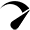# The Solution to CS50 psets 3 plurality problem (2022)CS50 psets 3 plurality problem solution

In this problem set, you must create a program that runs a plurality election. So, let’s see how you can approach this problem and do it.

When it comes to elections, they come in all shapes and sizes. The monarch officially appoints the prime minister of the UK. Generally, it is done by choosing the leader of the political party that wins the most seats in the house of commons. When it comes to the United States, they use a multi-step Electrol-College process where citizens vote on how each state should allocate electors who then elect the President using the results of the election.

There are many ways to hold an election. But what is the simplest way? The simplest way to hold an election is via a method called the “plurality vote”. It is also known as “first-past-the-post” or “winner-take-all”. In this method, every voter gets to vote for one candidate. So at the end of the election, the candidate who has the most votes is declared the winner of the election.

So, in this problem set, we have to complete the C code of the given plurality.c file. Here is the code, for the cs50 psets 3 plurality problem.

``````#include <cs50.h>
#include <stdio.h>
#include <string.h>

// Max number of candidates
#define MAX 9

// Candidates have name and vote count
typedef struct
{
string name;
}
candidate;

// Array of candidates
candidate candidates[MAX];

// Number of candidates
int candidate_count;

// Function prototypes
bool vote(string name);
void print_winner(void);

int main(int argc, string argv[])
{
// Check for invalid usage
if (argc < 2)
{
printf("Usage: plurality [candidate ...]\n");
return 1;
}

// Populate array of candidates
candidate_count = argc - 1;
if (candidate_count > MAX)
{
printf("Maximum number of candidates is %i\n", MAX);
return 2;
}
for (int i = 0; i < candidate_count; i++)
{
candidates[i].name = argv[i + 1];
}

int voter_count = get_int("Number of voters: ");

// Loop over all voters
for (int i = 0; i < voter_count; i++)
{
string name = get_string("Vote: ");

// Check for invalid vote
if (!vote(name))
{
printf("Invalid vote.\n");
}
}

// Display winner of election
print_winner();
}

// Update vote totals given a new vote
bool vote(string name)
{
// Loop through the count of candidates
for (int i = 0; i < candidate_count; i++)
{
// Check if the vote is similar to the name of the candidate
if (strcmp(candidates[i].name, name) == 0)
{
// if it is true then add a vote to the number of votes of that candidate
return true;
}
}
// else print invalid vote
return false;
}

// Print the winner (or winners) of the election
void print_winner(void)
{
// create a variable and set the value to 0
int num_vote = 0;

// iterate over the number of candidate
for (int i = 0; i < candidate_count; i++)
{
// check each and every vote and which one got the majority
{
}
}

// Iterate over the list of candidates
for (int i = 0; i < candidate_count; i++)
{
{
printf("%s\n", candidates[i].name);
}
}

return;
}``````

So, this is my solution to the cs50 psets 3 plurality problem.

You might also like to read: The solution to cs50 labs 3 sort problem (2022)

(Visited 63 times, 1 visits today)

### 2 ResponsesOptimized by Seraphinite Accelerator